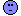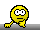ElMaestro
★★★

Denmark,
2021-08-04 08:18
(43 d 12:00 ago)

Posting: # 22502
Views: 1,469

## “Fixed Effects, Rather Than Random Effects…” [Regulatives / Guidelines]

Hi all,here's a paper that discusses the funky issue with fixed effects versus random effects.

Enjoy.
In particular, who can come up with a quantitative relevant measure of the difference any estimator makes if you have two model alternatives?

One thing is of course to judge if estimate A is closer to the true value that estimator B, or if its variance is smaller, but another is to judge practical relevance. I was told that it is the latter that is of interest to the author.Pass or fail!
ElMaestro
Helmut
★★★Vienna, Austria,
2021-08-04 10:16
(43 d 10:03 ago)

@ ElMaestro
Posting: # 22503
Views: 1,112

## Very nice!

Hi ElMaestro,

» Enjoy.

THX, I did.» In particular, who can come up with a quantitative relevant measure of the difference any estimator makes if you have two model alternatives?

Define relevant.» One thing is of course to judge if estimate A is closer to the true value that estimator B, or if its variance is smaller, …

$$\small{\delta_{\,estimate-true}\small}$$ or – likely better – $$\small{|\,\textrm{RE}\,(\%)\,|=|\,100(estimate-true)/true\,|}$$ is commonly used.
Example for $$\small{\sigma_\textrm{wR}^2=0.2025}$$ of the paper’s Table 2:
$$\textbf{Table I:}\;\textrm{Comparison of models' estimates}$$$$\small{\begin{array}{cccccccl} \hline \text{Scenario} & \hat\sigma_\textrm{wR,REML}^2 & \delta & |\,RE\,(\%)\,| & \hat\sigma_\textrm{wR,Lin.model}^2 & \delta & |\,RE\,(\%)\,| & \textrm{Comparison of }|\,RE\,(\%)\,|\\ \hline 1 & 0.2023 & -0.0002 & 0.0988 & 0.2024 & -0.0001 & 0.0494 & \text{Linear model "better"} \\ 2 & 0.2036 & +0.0011 & 0.5432 & 0.2036 & +0.0011 & 0.5432 & \text{Equal} \\ 3 & 0.2014 & -0.0011 & 0.5432 & 0.2013 & -0.0012 & 0.5926 & \text{REML "better"} \\ 4 & 0.2022 & -0.0003 & 0.1481 & 0.2023 & -0.0002 & 0.0988 & \text{Linear model "better"} \\ 5 & 0.2025 & \pm 0.0000 & 0.0000 & 0.2024 & -0.0001 & 0.0494 & \text{REML "better"} \\ 6 & 0.2027 & +0.0002 & 0.0988 & 0.2027 & +0.0002 & 0.0988 & \text{Equal} \\ \hline \end{array}}$$There is no clear winner. IMHO, it boils down to the question which of the scenarios is most likely occurring in practice. No idea.

We can look at the expanded limits $$\small{\left\{L\,,U\right\}}=100\exp(\mp0.76\,\hat{\sigma}_\textrm{wR})$$ and the back-calculated ‘clinically not relevant difference’ $$\small{\Delta^{\star}=100-L}$$ as well:
$$\textbf{Table II:}\;\textrm{Comparison of ABEL}$$$$\small{\begin{array}{ccccccc} \hline \text{Scenario} & \left\{\textit{L},\,\textit{U}\right\}_\textrm{REML} & \Delta^{\star} & \left\{\textit{L},\,\textit{U}\right\}_\textrm{LM} & \Delta^{\star} & \left\{\textit{L}-\textit{U}\right\} & \Delta^{\star} \\ \hline 1 & 71.05,\,140.75 & 28.95\% & 71.04,\,140.76 & 28.96\% & \textrm{REML}>\textrm{LM} & \textrm{REML}<\textrm{LM}\\ 2 & 70.97,\,140.91 & 29.03\% & 70.97,\,140.91 & 29.03\% & \textrm{REML}=\textrm{LM} & \textrm{REML}=\textrm{LM}\\ 3 & 70.10,\,140.65 & 28.90\% & 71.11,\,140.63 & 28.89\% & \textrm{REML}>\textrm{LM} & \textrm{REML}>\textrm{LM}\\ 4 & 71.05,\,140.74 & 28.95\% & 71.05,\,140.75 & 28.95\% & \textrm{REML}=\textrm{LM} & \textrm{REML}=\textrm{LM}\\ 5 & 71.03,\,140.78 & 28.97\% & 71.04,\,140.76 & 28.96\% & \textrm{REML}>\textrm{LM} & \textrm{REML}>\textrm{LM}\\ 6 & 71.02,\,140.80 & 28.98\% & 71.02,\,140.80 & 28.98\% & \textrm{REML}=\textrm{LM} & \textrm{REML}=\textrm{LM}\\ \hline \end{array}}$$A sponsor would prefer $$\small{\left\{L\,,U\right\}}$$ as wide as possible. Hence, $$\small{\textrm{REML}}$$ is the way to go. From the patient’s – and therefore, regulatory? – perspective it is obviously the other way ’round $$\small{(\Delta^{\star}}$$ as small as possible). Bonus question: What about the Type I Error? Of course, discussed in the paper…

» … but another is to judge practical relevance. I was told that it is the latter that is of interest to the author.Understandable. However, even if not practically relevant I prefer the “better” one. The paper’s Table 4 is interesting.

Dif-tor heh smusma 🖖
Helmut SchützThe quality of responses received is directly proportional to the quality of the question asked. 🚮
Science Quotes
ElMaestro
★★★

Denmark,
2021-08-04 12:51
(43 d 07:28 ago)

@ Helmut
Posting: # 22504
Views: 1,074

## Very nice!

Hi Hötzi,

» Define relevant.Please go right ahead help me define it.Relevance is not part of the inventory in my department.One thing that is not explicit in the paper but which is really funny is that:
1. The individual treatment estimates are completely, utterly, terribly off with the lm.
2. The individual treatment estimates are very close to true with the mm.
3. Both give the same treatment differences as you can see in the paper.

I'd like to study the phenomenon a bit more with e.g. datasets having missing values. But on my old toaster these things run slow.

Bonus: Someone tell me how to control bobyqa convergence in a paradigm that fits into things like tolerance or relative tolerance. I don't quite get the convergence principles for that optimzer. It would help speed up experiments.

Pass or fail!
ElMaestro
Helmut
★★★Vienna, Austria,
2021-08-04 15:22
(43 d 04:56 ago)

@ ElMaestro
Posting: # 22505
Views: 1,045

## Narrower CI with LM?

Hi ElMaestro,

» » Define relevant.»
» Please go right ahead help me define it.» Relevance is not part of the inventory in my department.In mine not either…

» One thing that is not explicit in the paper but which is really funny is that:
» 1. The individual treatment estimates are completely, utterly, terribly off with the lm.
» 2. The individual treatment estimates are very close to true with the mm.
» 3. Both give the same treatment differences as you can see in the paper.

#1 & #2 hints towards the mixed model. #3 is interesting – meaning out?

BTW, from the Introduction:

EU regulators did not present arguments for their proposal, and at the time of introduction they expressed that their approach is straightforward to calculate.

Exactly. [But unsubstantiated.] Made up out of thin air.

I still think that the “all effects fixed” approach is questionable, at least. It assumes homoscedasticity of within-subject variances $$\small{(\sigma_\textrm{wT}^2\equiv\sigma_\textrm{wR}^2})$$, a condition, which is – more often than not – outright false. I have seen many full replicate studies, where $$\small{s_\textrm{wT}^2<s_\textrm{wR}^2}$$. Rarely it was the other way ’round. Biopharmaceutical technology improves.

Of note, a mixed-model is mandatory for the FDA1,2 and Health Canada3 writes:

By definition the cross‐over design is a mixed effects model with fixed and random effects.

(my emphasis)

The confidence interval of the mixed-effects model can be wider than the one of the Linear Model (LM). It depends on how much information can be recovered and moved to the additional terms (in the LM all remains in the residual error). If little can be recovered, the CI of the mixed-effects model will be wider due to less degrees of freedom. Even observed in theomniscient oracle’s fabricated data sets.4
$$\small{\begin{array}{ccrcccc} \hline \text{Data set} & \text{Model} & df & t_\textrm{crit} & \text{PE} & \text{90% CI} & \textrm{Width} \\ \hline \text{I } & \text{LM} & 217.0 & 1.6519 & 115.65{\color{red}9} & \text{107.106, 124.895} & {\color{Red}{17.789\%}} \\ \text{I } & \text{REML} & 207.7 & 1.6522 & 115.65{\color{red}8} & \text{107.104, 124.894} & {\color{DarkGreen}{17.790\%}} \\ \hline \text{II} & \text{LM} & 45.00 & 1.6794 & 102.264 & \text{ 97.316, 107.465} & {\color{Red}{10.149\%}} \\ \text{II} & \text{REML} & 19.89 & 1.7252 & 102.264 & \text{ 97.053, 107.755} & {\color{DarkGreen}{10.702\%}} \\\hline \end{array}}$$
» I'd like to study the phenomenon a bit more with e.g. datasets having missing values. But on my old toaster these things run slow.

Can imagine.
Even the point estimates might be different, which is not so obvious in ‘data set I’ with missings above. In one of my studies (partial replicate, unbalanced: $$\small{n_1=n_2=21}$$, $$\small{n_3=20}$$ and incomplete: one missing in the 2nd period and seven in the 3rd) I got:
$$\small{ \begin{array}{cccccc} \hline \text{Model} & df & t_\textrm{crit} &\text{PE} & \text{90% CI} & \textrm{Width} \\ \hline \text{LM} & 113.00 & 1.6585 & 107.194 & \text{98.307, 116.884} & 18.577 \\ \text{REML} & \text{ 57.81} & 1.6716 & 106.933 & \text{98.616, 115.951} & 17.335 \\\hline \end{array}}$$In this case information could be recovered and hence, the confidence interval of REML was narrower than the LM’s, despite its fewer degrees of freedom.

Endrényi and Tóthfalusi noted5 that this lack of harmonization of statistical approaches might lead – in the hypothetical situation of submitting the same study to different agencies – to acceptance in one jurisdiction and rejection in another. See also a desperate attempt from the real world.

» Bonus: Someone tell me how to control bobyqa convergence …

Sorry, never met uncle Bob in person.

1. FDA (CDER). Guidance for Industry. Statistical Approaches to Establishing Bioequivalence. Rockville. January 2001. download.
2. FDA (OGD). Draft Guidance on Progesterone. Recommended Apr 2010; Revised Feb 2011. download.
3. Health Canada. Guidance Document. Conduct and Analysis of Comparative Bioavailability Studies. Ottawa. Revised 2018/06/08. Section 2.7.4.2 Model fitting. download.
4. EMA. Clinical pharmacology and pharmacokinetics: questions and answers. 3.1 Which statistical method for the analysis of a bioequivalence study does the Agency recommend? Annex I. London. 21 September 2016. EMA/582648/2016.
5. Endrényi L, Tóthfalusi. Bioequivalence for highly variable drugs: regulatory agreements, disagreements, and harmonization. J Pharmacokin Pharmacodyn. 2019; 46(2): 117–26. doi:10.1007/s10928-019-09623-w.

Dif-tor heh smusma 🖖
Helmut SchützThe quality of responses received is directly proportional to the quality of the question asked. 🚮
Science QuotesIng. Helmut Schütz